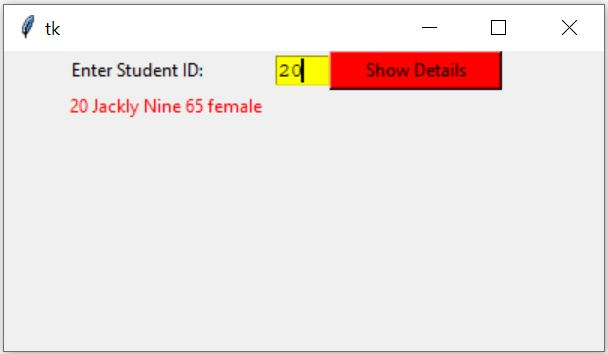# Displaying record from MySQL table based on user entered ID

Basics of Python Tkinter

Display records from MySQL Student table in Tkinter window by using entered ID of the row.

Tkinter window to display row details from MySQL table based on the user input of record id## Connect to MySQL database

We connected to MySQL database by using our userid, password and database name. You can update your MySQL login details here.
``````import mysql.connector

my_connect = mysql.connector.connect(
host="localhost",
user="userid",
database="database_name"
)
####### end of connection ####
my_cursor = my_connect.cursor()``````
We will use my_cursor in our further script as the connection object to get our records.

## Adding components to tkinter

First add t1 the Text box to receive student id from the user. This id we will use to collect matching record from the student table.
We will add one Lebel l1 to display text Enter Student ID: before the text box t1.
``````# add one Label
l1 = tk.Label(my_w,  text='Enter Student ID: ', width=25 )
l1.grid(row=1,column=1)

# add one text box
t1 = tk.Text(my_w,  height=1, width=4,bg='yellow')
t1.grid(row=1,column=2) ``````
We need one more Lebel ( l2) to display the returned details of the student. This Label initially will display the string Output.
We will be displaying data as we are getting from MySQL table, so we will declare one tkinter string variable ( StringVar() ) connected to our Label. We declared my_str = tk.StringVar() for this.
``````my_str = tk.StringVar()
# add one Label
l2 = tk.Label(my_w,  textvariable=my_str, width=30,fg='red' )
l2.grid(row=3,column=1,columnspan=2)

my_str.set("Output") # assigning string data``````
We will add one Button b1 to pass user entered data to a function to get the record details.

## click event of the button b1

Once the Button is clicked it will read the data entered in the text box t1 and pass it to the user defined function my_details().
``````b1 = tk.Button(my_w, text='Show Details', width=15,bg='red',
command=lambda: my_details(t1.get('1.0',END)))
b1.grid(row=1,column=4)``````

## my_details()

On click of the Button b1 , my_details() function will receive the string id as input parameter. This is the id of the student for which all the detail of the record will be taken from student table and displayed by using l2 label.

Here data ( id ) is entered by user through the text field ( t1 ). So before using this data in our database Query, we must check this input data.
Inside the function my_details() we will use try except code blocks to check if the user entered data id is Integer or not. If it is not integer then except: part of the code block will display message Check input.
`````` try:
val = int(id) # check input is integer or not
except:
my_str.set("Check input")``````
Inside the try block ( the input id is integer ) we will keep one more try and except. In this try block we will keep the database handling part and in except block we will display message Database error. The full code of the function my_details() is here.
``````def my_details(id):
try:
val = int(id) # check input is integer or not
try:
my_cursor.execute("SELECT * FROM student WHERE id="+id)
student = my_cursor.fetchone()
#print(student)
my_str.set(student)

except :
my_str.set("Database error")
except:
my_str.set("Check input")``````
We used SQL with WHERE to collect details of the record by using student id from the student table.

Note that MySQL database executes the query part only and return us one result set. This result set contains details of our row data of input ID.
We used the returned MySQLCursor i.e my_cursor
The full code is here , Change the userid,password and database name of your MySQL database.
``````import mysql.connector
import tkinter  as tk
from tkinter import *
my_connect = mysql.connector.connect(
host="localhost",
user="userid",
database="database_name"
)
my_cursor = my_connect.cursor()
####### end of connection ####

my_w = tk.Tk()
my_w.geometry("400x200")

# add one Label
l1 = tk.Label(my_w,  text='Enter Student ID: ', width=25 )
l1.grid(row=1,column=1)

# add one text box
t1 = tk.Text(my_w,  height=1, width=4,bg='yellow')
t1.grid(row=1,column=2)

b1 = tk.Button(my_w, text='Show Details', width=15,bg='red',
command=lambda: my_details(t1.get('1.0',END)))
b1.grid(row=1,column=4)

my_str = tk.StringVar()
# add one Label
l2 = tk.Label(my_w,  textvariable=my_str, width=30,fg='red' )
l2.grid(row=3,column=1,columnspan=2)

my_str.set("Output")

def my_details(id):
try:
val = int(id) # check input is integer or not
try:
my_cursor.execute("SELECT * FROM student WHERE id="+id)
student = my_cursor.fetchone()
#print(student)
my_str.set(student)

except :
my_str.set("Database error")
except:
my_str.set("Check input")
my_w.mainloop()``````
Using SQLAlchemy full code is here
``````import tkinter  as tk
from tkinter import *
my_w = tk.Tk()
my_w.geometry("400x250")

l1 = tk.Label(my_w,  text='Enter Student ID: ', width=25 )
l1.grid(row=1,column=1)

t1 = tk.Text(my_w,  height=1, width=4,bg='yellow')
t1.grid(row=1,column=2)

b1 = tk.Button(my_w, text='Show Details', width=15,bg='red',
command=lambda: my_details(t1.get('1.0',END)))
b1.grid(row=1,column=4)

my_str = tk.StringVar()
my_str.set('Output here ')
# add one Label
l2 = tk.Label(my_w,  textvariable=my_str, width=30,fg='red' )
l2.grid(row=3,column=1,columnspan=2)
from sqlalchemy import create_engine
from sqlalchemy.exc import SQLAlchemyError

def my_details(id):
try:
val = int(id) # check input is integer or not
try:
my_data=(val,)
my_row=my_conn.execute("SELECT * FROM student WHERE id=%s",my_data)
student = my_row.fetchone()
#print(student)
my_str.set(student)

except :
my_str.set("Database error")
except:
my_str.set("Check input")

my_w.mainloop()``````

Subscribe to our YouTube Channel here

## Subscribe

* indicates required
Subscribe to plus2netplus2net.com

Post your comments , suggestion , error , requirements etc here